# Midterm Review CS 5010 Program Design Paradigms Bootcamp

• Slides: 17Introduction • This lesson is a review of the main points of the first part of this course. • These are mostly slides you should remember, or remixes of some of those slides. • By the end of this lesson, you should be able to give at least two strategies for choosing a design strategy. 2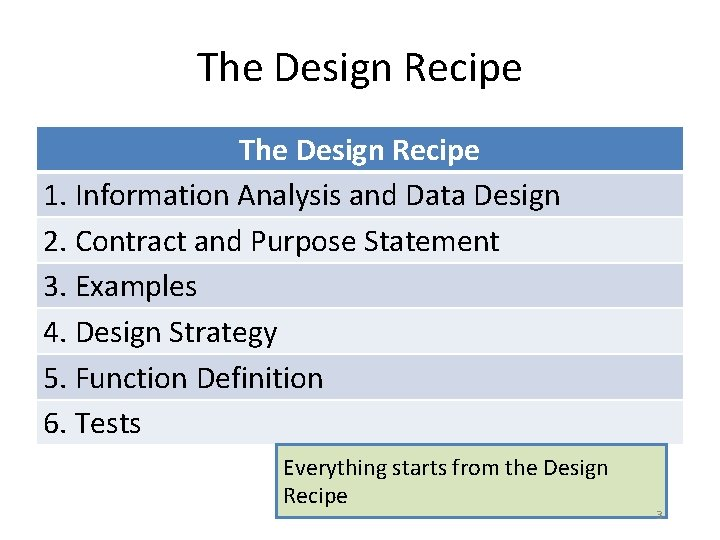The Design Recipe 1. Information Analysis and Data Design 2. Contract and Purpose Statement 3. Examples 4. Design Strategy 5. Function Definition 6. Tests Everything starts from the Design Recipe 3Design functions systematically • Follow the recipe! • The structure of the data tells you the structure of the program. – Or at least gives you good hints! – Data Definition Template Code – The data definitions structure your wishlist, too. • Examples make you clarify your thinking – Be sure to cover corner cases Here are three of our favorite slogans 4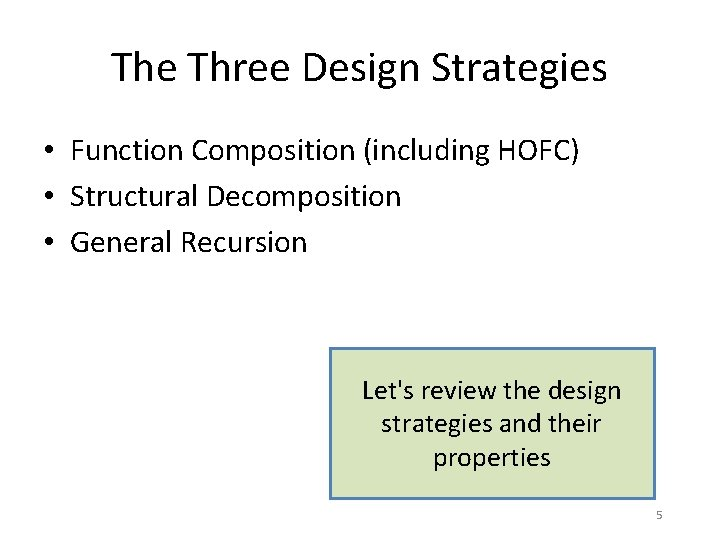The Three Design Strategies • Function Composition (including HOFC) • Structural Decomposition • General Recursion Let's review the design strategies and their properties 5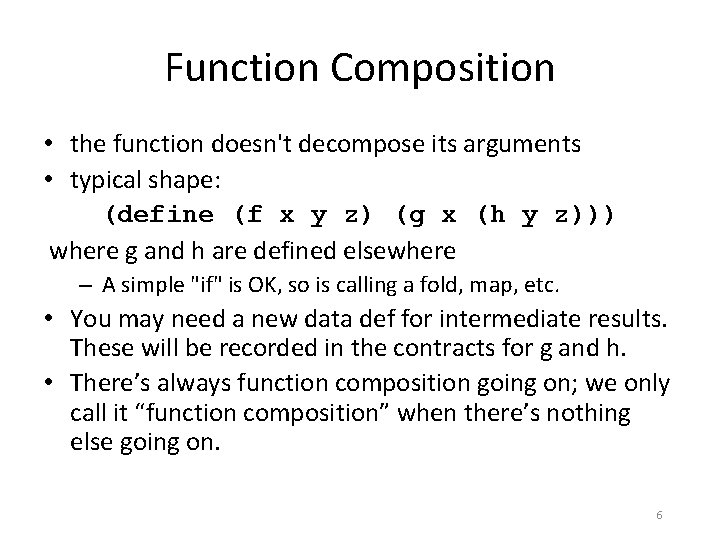Function Composition • the function doesn't decompose its arguments • typical shape: (define (f x y z) (g x (h y z))) where g and h are defined elsewhere – A simple "if" is OK, so is calling a fold, map, etc. • You may need a new data def for intermediate results. These will be recorded in the contracts for g and h. • There’s always function composition going on; we only call it “function composition” when there’s nothing else going on. 6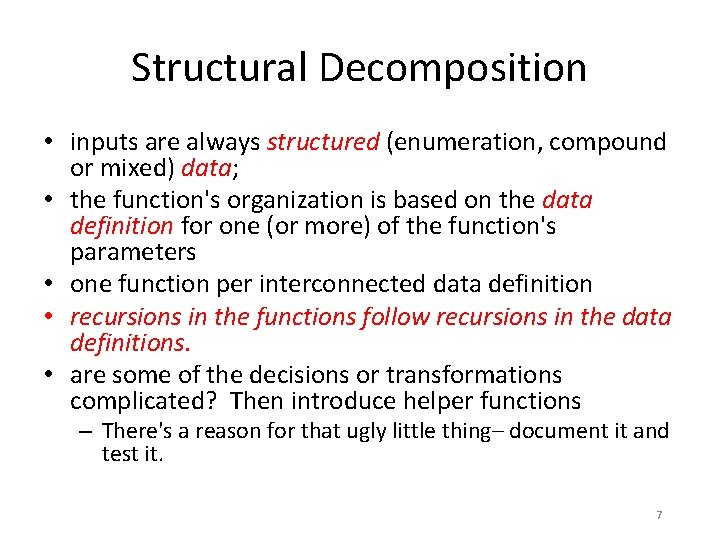Structural Decomposition • inputs are always structured (enumeration, compound or mixed) data; • the function's organization is based on the data definition for one (or more) of the function's parameters • one function per interconnected data definition • recursions in the functions follow recursions in the data definitions. • are some of the decisions or transformations complicated? Then introduce helper functions – There's a reason for that ugly little thing– document it and test it. 7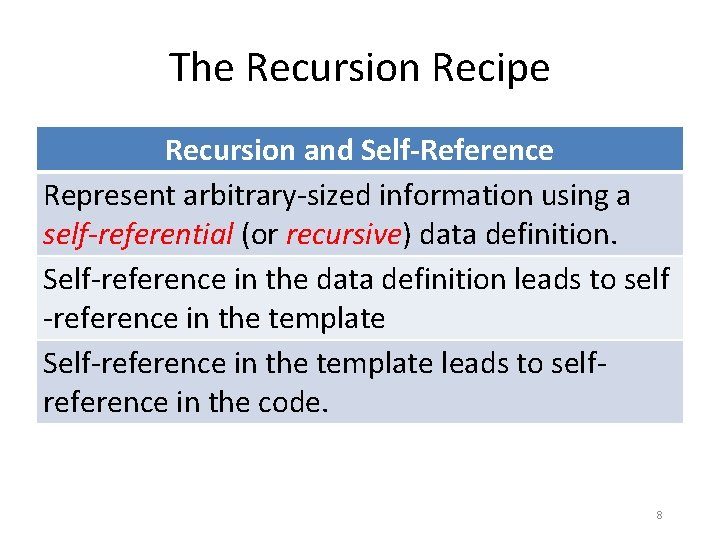The Recursion Recipe Recursion and Self-Reference Represent arbitrary-sized information using a self-referential (or recursive) data definition. Self-reference in the data definition leads to self -reference in the template Self-reference in the template leads to selfreference in the code. 8General Recursion • Inputs encode problems from a class of problems • Recursion solves a related problem from the same class (“subgoal” or “subproblem”) – requires ad hoc insight to find a useful subproblem. • Termination argument is required: – how are each of the subproblems easier than the original problem? – formulate this as a halting measure. 9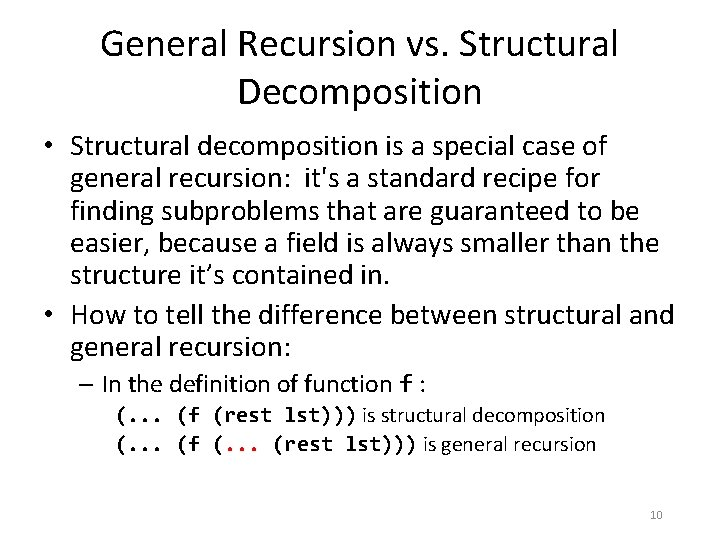General Recursion vs. Structural Decomposition • Structural decomposition is a special case of general recursion: it's a standard recipe for finding subproblems that are guaranteed to be easier, because a field is always smaller than the structure it’s contained in. • How to tell the difference between structural and general recursion: – In the definition of function f : (. . . (f (rest lst))) is structural decomposition (. . . (f (. . . (rest lst))) is general recursion 10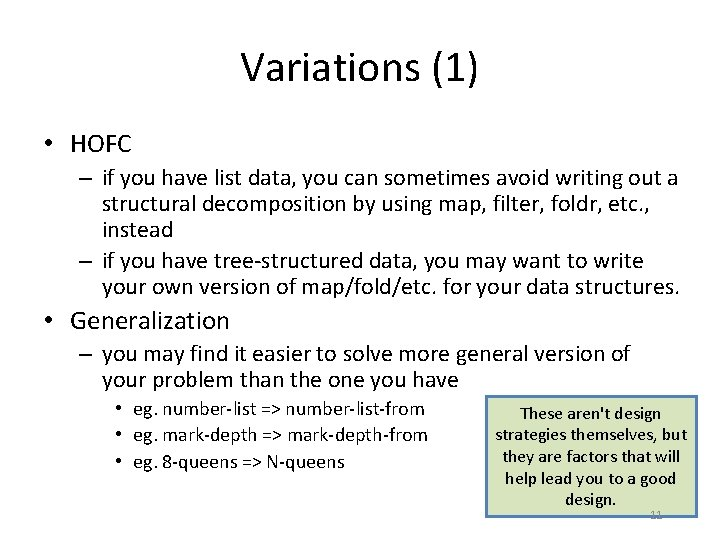Variations (1) • HOFC – if you have list data, you can sometimes avoid writing out a structural decomposition by using map, filter, foldr, etc. , instead – if you have tree-structured data, you may want to write your own version of map/fold/etc. for your data structures. • Generalization – you may find it easier to solve more general version of your problem than the one you have • eg. number-list => number-list-from • eg. mark-depth => mark-depth-from • eg. 8 -queens => N-queens These aren't design strategies themselves, but they are factors that will help lead you to a good design. 11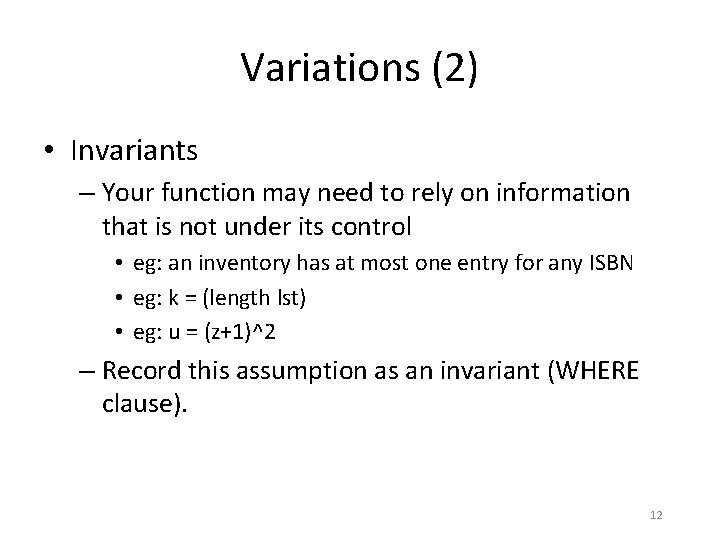Variations (2) • Invariants – Your function may need to rely on information that is not under its control • eg: an inventory has at most one entry for any ISBN • eg: k = (length lst) • eg: u = (z+1)^2 – Record this assumption as an invariant (WHERE clause). 12Choosing a Design Strategy • If the problem is trivial, then the solution should be trivial: use function composition. • If the problem is non-trivial, then reduce the problem to one or more simpler problems, and reconstruct the solution to your problem from the solutions to the simpler problems OK, those are the possible design strategies. How do we choose among them? 13Finding the Simpler Subproblems • If there are independent/sequential pieces, use functional composition • Otherwise, find one or more simpler instances of same problem: – Is the simpler instance a substructure of the original? If so, use structural decomposition (maybe w/ accumulator) – Otherwise, use general recursion. You've been doing this all term, so you probably know this. But it's worth writing 14 down anyway.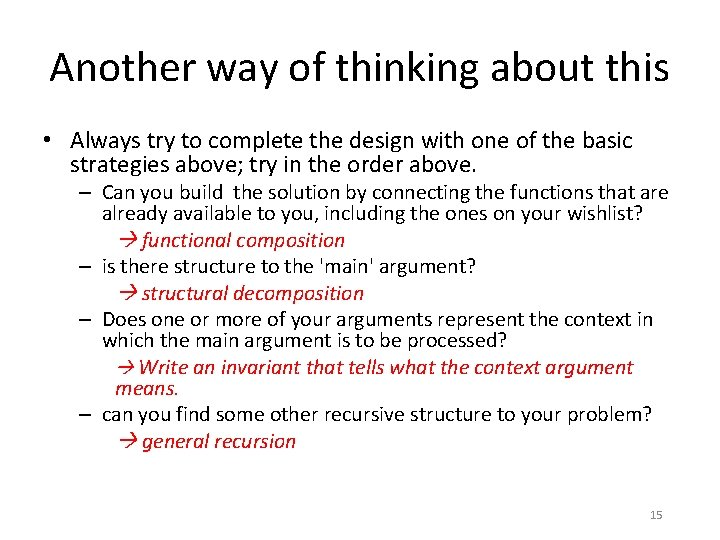Another way of thinking about this • Always try to complete the design with one of the basic strategies above; try in the order above. – Can you build the solution by connecting the functions that are already available to you, including the ones on your wishlist? functional composition – is there structure to the 'main' argument? structural decomposition – Does one or more of your arguments represent the context in which the main argument is to be processed? Write an invariant that tells what the context argument means. – can you find some other recursive structure to your problem? general recursion 15Summary • We've reviewed the big take-away points from the first half of the course. • Next: we will move on to classes and objects. 16